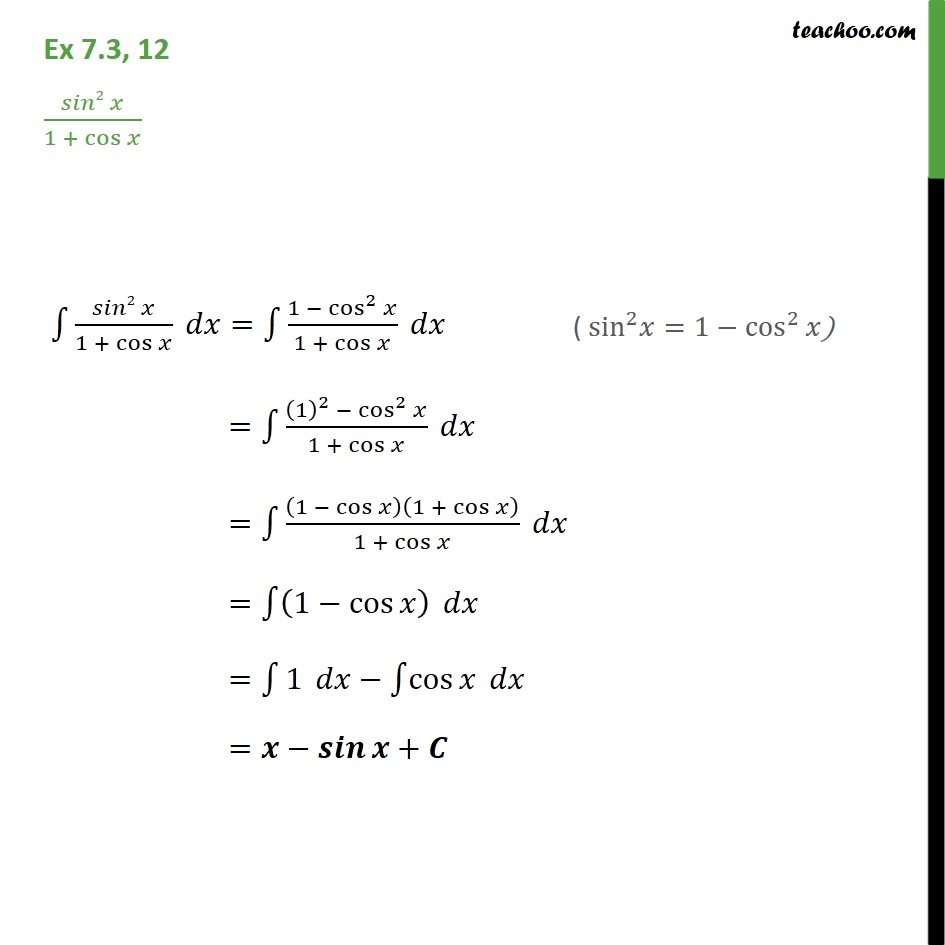Integration Full Chapter Explained - Integration Class 12 - Everything you need1. Chapter 7 Class 12 Integrals
2. Serial order wise
3. Ex 7.3

Transcript

Ex 7.3, 12 ( 2 )/(1 + cos ) 1 ( 2 )/(1 + cos ) = 1 (1 cos^2 )/(1 + cos ) = 1 ((1)^2 cos^2 )/(1 + cos ) = 1 (1 cos )(1 + cos )/(1 + cos ) = 1 (1 cos ) = 1 1 1 cos = +

Ex 7.3

Chapter 7 Class 12 Integrals
Serial order wise